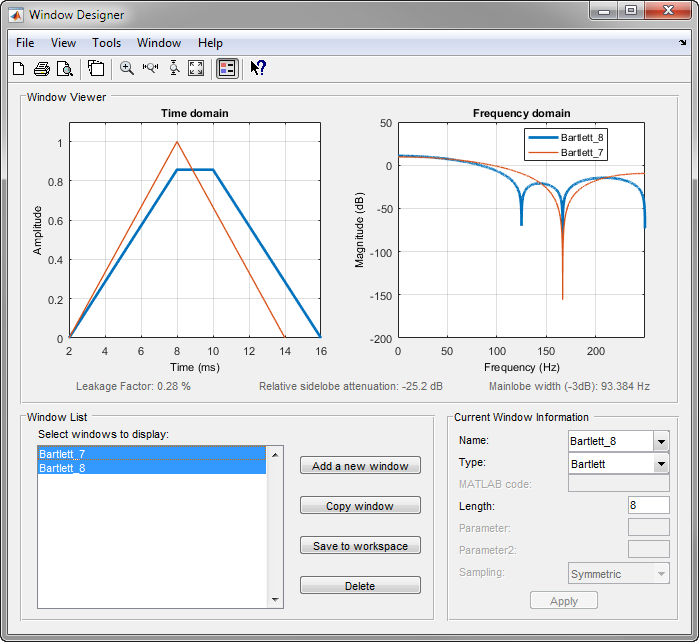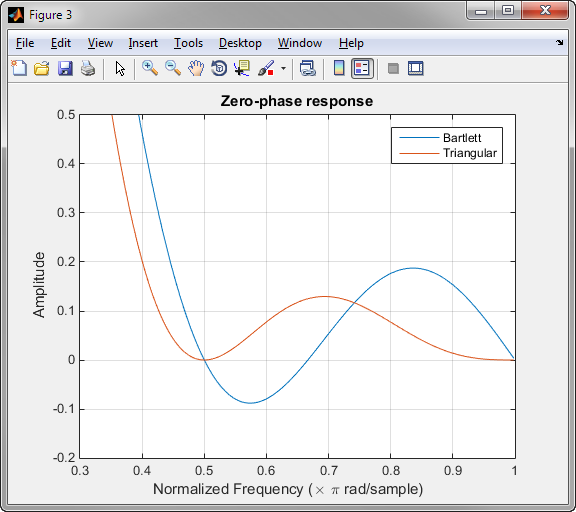## Windows

### Why Use Windows?

In both digital filter design and spectral estimation, the choice of a windowing function can play an important role in determining the quality of overall results. The main role of the window is to damp out the effects of the Gibbs phenomenon that results from truncation of an infinite series.

### Available Window Functions

Window

Function

Bartlett-Hann window

Bartlett window

Blackman window

Blackman-Harris window

Bohman window

Chebyshev window

Flat Top window

`flattopwin`

Gaussian window

`gausswin`

Hamming window

`hamming`

Hann window

`hann`

Kaiser window

`kaiser`

Nuttall's Blackman-Harris window

`nuttallwin`

Parzen (de la Vallée-Poussin) window

`parzenwin`

Rectangular window

`rectwin`

Tapered cosine window

`tukeywin`

Triangular window

`triang`

### Graphical User Interface Tools

Two graphical user interface tools are provided for working with windows in the Signal Processing Toolbox™ product:

• Window Visualization Tool (WVTool)

Refer to the reference pages for detailed information.

### Basic Shapes

The basic window is the rectangular window, a vector of ones of the appropriate length. A rectangular window of length 50 is

```n = 50; w = rectwin(n); ```

This toolbox stores windows in column vectors by convention, so an equivalent expression is

```w = ones(50,1); ```

To use the Window Designer app to create this window, type

```windowDesigner ```

The app opens with a default Hamming window. To visualize the rectangular window, set Type = Rectangular and Length = 50 in the Current Window Information panel and then press .

The Bartlett (or triangular) window is the convolution of two rectangular windows. The functions `bartlett` and `triang` compute similar triangular windows, with three important differences. The `bartlett` function always returns a window with two zeros on the ends of the sequence, so that for `n` odd, the center section of `bartlett(n+2)` is equivalent to `triang(n)`:

```Bartlett = bartlett(7); isequal(Bartlett(2:end-1),triang(5))```
```ans = 1```

For `n` even, `bartlett` is still the convolution of two rectangular sequences. There is no standard definition for the triangular window for `n` even; the slopes of the line segments of the `triang` result are slightly steeper than those of `bartlett` in this case:

```w = bartlett(8); [w(2:7) triang(6)] ```

You can see the difference between odd and even Bartlett windows in Window Designer.The final difference between the Bartlett and triangular windows is evident in the Fourier transforms of these functions. The Fourier transform of a Bartlett window is negative for `n` even. The Fourier transform of a triangular window, however, is always nonnegative.

The following figure, which plots the zero-phase responses of 8-point Bartlett and Triangular windows, illustrates the difference.

```zerophase(bartlett(8)) hold on zerophase(triang(8)) legend('Bartlett','Triangular') axis([0.3 1 -0.2 0.5])```This difference can be important when choosing a window for some spectral estimation techniques, such as the Blackman-Tukey method. Blackman-Tukey forms the spectral estimate by calculating the Fourier transform of the autocorrelation sequence. The resulting estimate might be negative at some frequencies if the window's Fourier transform is negative.